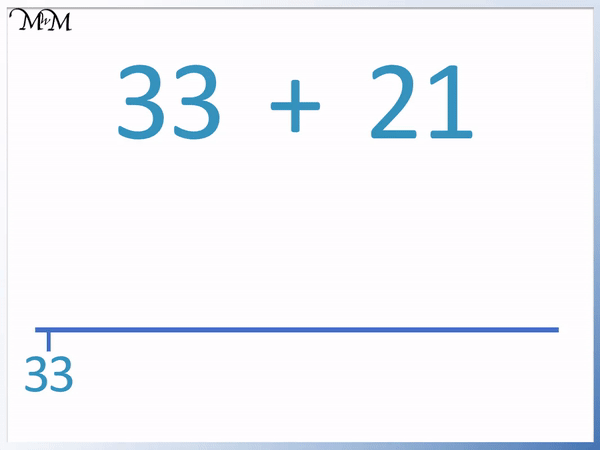• To add numbers with the jump strategy first draw a blank number line.
• Mark the largest number on the left of the number line.
• 33 is larger than 21 so we mark 33.
• We break the addition down into tens and units.
• 21 has 2 tens and 1 unit.
• We add the two tens first by drawing two jumps of 10.
• 10 is easy to add because the units digit remains the same but we increase the tens digit by 1.
• 33 + 10 = 43 and then 43 + 10 = 53.
• We now show the addition of the 1 unit with a smaller jump.
• The jump strategy is used to break down an addition and show the process visually.

Make jumps in tens and then ones to break down the addition.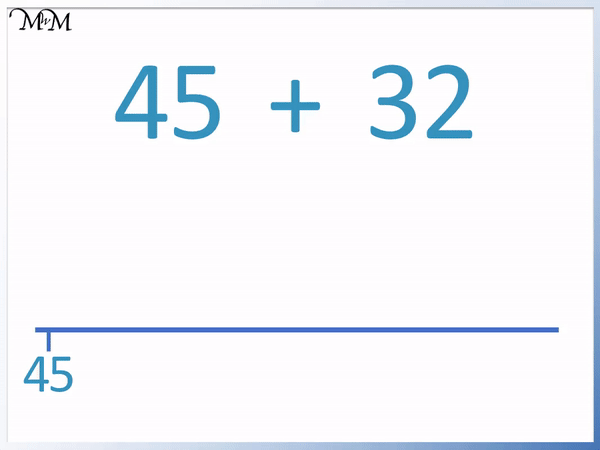• 45 is the larger number so we mark it on the left of the blank number line.
• 32 contains 3 tens.
• We can add 3 tens in one go by increasing the tens digit by 3.
• 4 + 3 = 7 and so, 45 + 30 = 75.
• We draw an arrow jump to the right on our number line from 45 to 75.
• 32 contains 2 units.
• 5 + 2 = 7 and so, 75 + 2 = 77.
• We draw a smaller jump from 75 to 77 on the number line.# The Jump Strategy for Addition

## What is the Jump Strategy for Addition?

The jump strategy is a visual method in which an addition is performed by adding the tens and ones separately in jumps. A blank number line is drawn and each addition is shown using arrow jumps.

Here is an example of an addition that will be performed using the jump strategy.

We have 33 + 21.

The jump strategy is also known as the blank number line strategy or empty number line strategy. This is because we start with a blank number line.We can see that we split the addition of 21 into adding 2 tens and 1 one. When teaching the jump strategy, it is important to understand how numbers can be partitioned into tens and ones.

We show the addition of the 2 tens with two separate jumps of 10 on our number line.

33 + 10 = 43

43 + 10 = 53

When teaching the jump strategy, we use these jumps to reinforce how the addition is broken down. We have 2 tens in 21 and so we have two jumps.

53 + 1 = 54 We show the addition of 1 using a smaller jump than the addition of 10.

When first introducing the jump strategy for addition, we can refer back to the number itself and show how it corresponds to the number of jumps that we have.

21 contains 2 jumps of ten and 1 jump of 1.

As you become more fluent with the strategy, we can reduce the method to only 2 jumps.

We have the same example, this time making the jump of 20 in one go.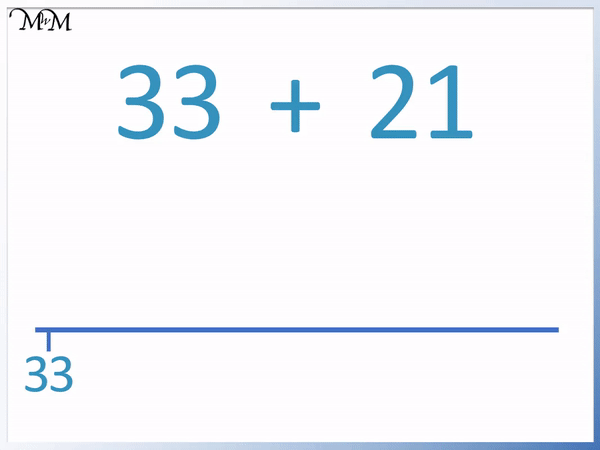Instead of adding 2 jumps of 10, we can add 20 in one go. This is quicker and takes up less space on the number line.

The jump strategy is used to break down an addition into separate chunks. The jump strategy is useful because it provides a visual picture for the addition.

It is easy to see how many parts of the number we have added because each addition is shown as an extra jump.

The number line helps to provide a frame of reference to see how the size of the answer compares with the original number.

Whilst it is not the most efficient method for addition, the process can help to build understanding of addition, number size and partitioning.

## Teaching Addition using the Jump Strategy

To add using the jump strategy, use the following steps:

• Draw an empty number line.
• Mark the largest number in the addition on the left side of this line.
• Look at the tens digit of the smaller number and add this digit to the tens digit of the larger number.
• Draw an arrow from the larger number to the right and write the answer to this addition below it.
• Look at the ones digit of the smaller number and add this digit to the ones digit of the larger number.
• Draw an arrow from the previous answer to the right and write this new answer below it.

We will use these steps to add 45 + 32 using the jump strategy.

We draw an empty number line.

45 is larger than 32, so we start with 45 marked on the left of this number line.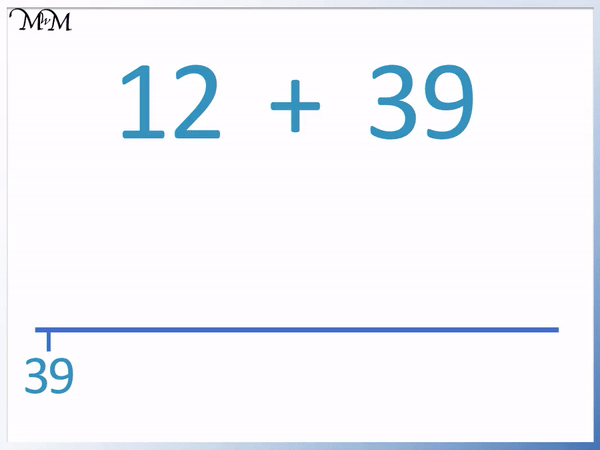32 contains 3 tens and 2 units. 3 tens is 30.

We draw an arrow from 45 to the right.

Adding tens is easy because the units digit remains the same. We just increase the tens column. To add 3 tens, we add 3 to the 4 tens in 45.

4 + 3 = 7 and so, 45 + 30 = 75.

We can see that the tens digit increased by 3 from 4 to 7. The units digit remained as 5.

We then add the 2 ones.

75 + 2 = 77

Therefore 45 + 32 = 77.

We can also add the 3 tens as 3 individual jumps of 10 and then add the 2 ones as 2 individual jumps of one.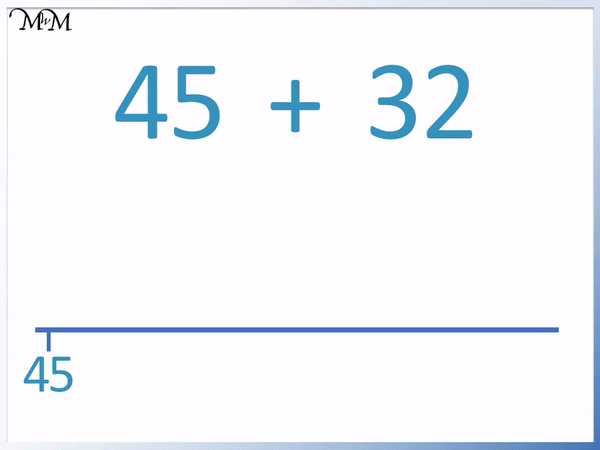Here is another example of addition using a blank number line.

We have 12 + 39.

39 is larger than 12 and so, we start with 39 marked on the left of the number line.

The reason for this is that it is easier to add 12 to 39 than it is to add 39 to 12.

Jumps to add 12 is shorter and involves smaller numbers than jumps which as 39.12 contains just 1 ten and 2 ones.

39 + 10 = 49

Adding ten is easy because both numbers end in 9 and we simply increase the 3 to a 4.

We could add the 2 ones in one go, however 9 + 2 = 11. Additions that cross a tens value are typically more difficult.

49 + 2 = 51 but it can be easier to show this as two separate jumps of 1.

When teaching the jump strategy, it is important to be able to choose between jumping in separate, many groups of ten or one and jumping all of the tens or ones in one go.Now try our lesson on Addition using the Compensation Strategy where we learn how to use the compensation strategy to add two numbers.